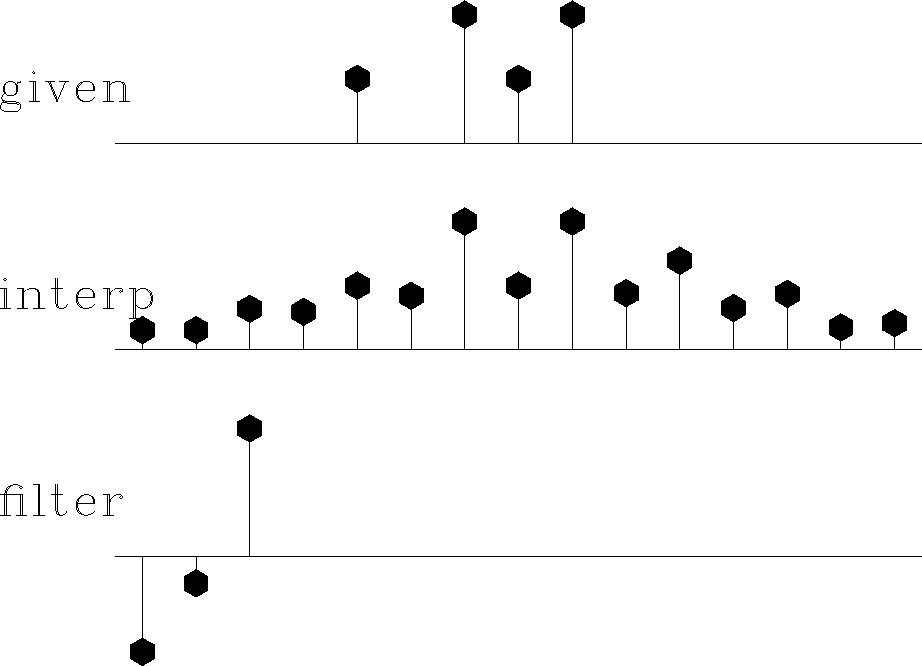Next: Objections to interpolation error Up: Missing-data restoration Previous: Missing-data program

# MISSING DATA AND UNKNOWN FILTER

Recall the missing-data figures beginning with Figure 2. There the filters were taken as known, and the only unknowns were the missing data. Now, instead of having a predetermined filter, we will solve for the filter along with the missing data. The principle we will use is that the output power is minimized while the filter is constrained to have one nonzero coefficient (else all the coefficients would go to zero). We will look first at some results and then see how they were found.

In Figure 8 the filter is constrained to be of the form (1,a1,a2).

 missif Figure 8 Top is known data. Middle includes the interpolated values. Bottom is the filter with the leftmost point constrained to be unity and other points chosen to minimize output power.The result is pleasing in that the interpolated traces have the same general character as the given values. The filter came out slightly different from the (1,0,-1) that I suggested for Figure 7 based on a subjective analysis. Curiously, constraining the filter to be of the form (a-2,a-1,1) in Figure 9 yields the same interpolated missing data as in Figure 8. I understand that the sum squared of the coefficients of A(Z)P(Z) is the same as that of A(1/Z)P(Z), but I do not see why that would imply the same interpolated data.

 backwards Figure 9 The filter here had its rightmost point constrained to be unity--i.e., this filtering amounts to backward prediction. The interpolated data seems to be identical, as with forward prediction.Next: Objections to interpolation error Up: Missing-data restoration Previous: Missing-data program
Stanford Exploration Project
10/21/1998